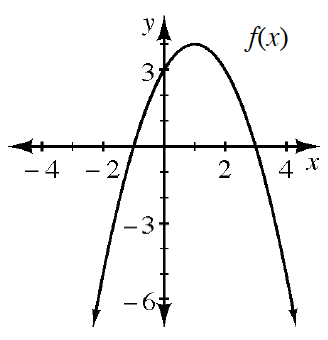### Home > CCA > Chapter 11 > Lesson 11.3.1 > Problem11-82

11-82.

Find the equation of $f(x)$ graphed below.Find the $x$-intercepts.
Their $x$-coordinates are roots of the equation.

See the homework help for problems 11-13 and 11-69.

Use the eTool below to help you find the equation for each function.
Click on the link at right for the full eTool version: CCA 11-82 HW eTool